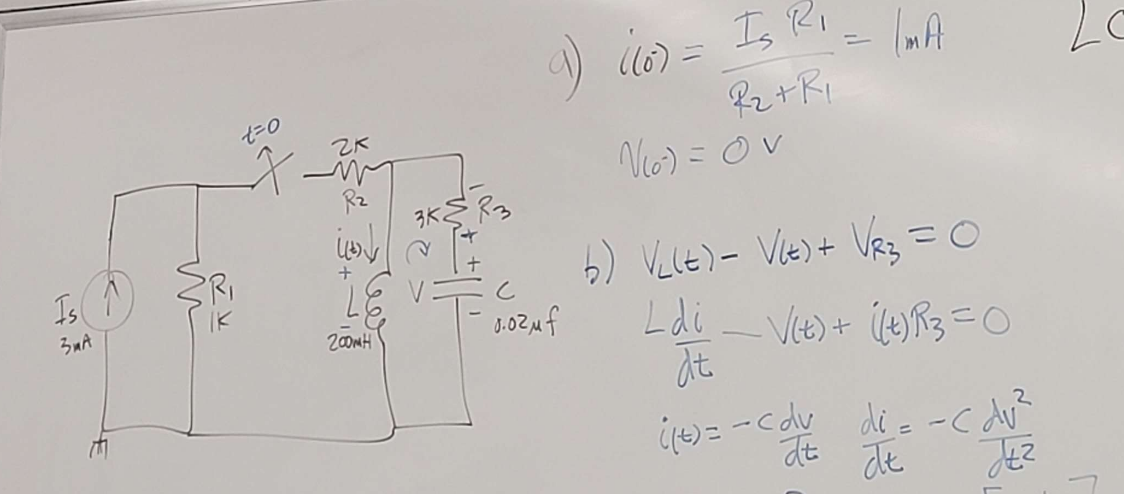# (Solved): Just had a clarification question. I understand why the i0 is 1 mA (current divider rule), but why ...Just had a clarification question. I understand why the i0 is 1 mA (current divider rule), but why is v0 = 0V? My thinking is that the voltage across R2 is equal to the voltage across the cap.

b)

We have an Answer from Expert